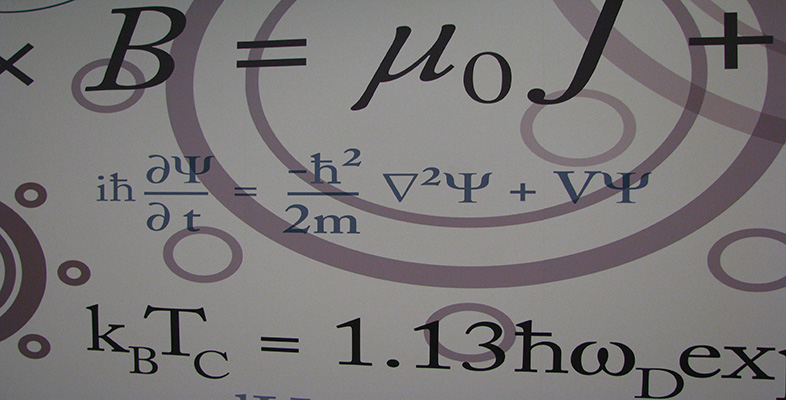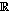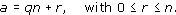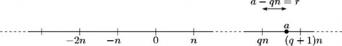Number systems

This free course is available to start right now. Review the full course description and key learning outcomes and create an account and enrol if you want a free statement of participation.

Free course

3 Modular arithmetic

3.1 Division

In this section, instead of enlarging the number system, we do arithmetic with finite sets of integers, namely the sets of possible remainders when we divide by particular positive integers. This type of arithmetic is important in number theory (the study of the integers) and in cryptography. It is used frequently in group theory.

If we divide one positive integer by another we obtain a quotient and a remainder. For example, 29 divided by 4 gives quotient 7 and remainder 1 because 29 = 7 × 4 + 1. If we divide any positive integer by 4, the remainder will be one of the numbers 0, 1, 2, 3.

This idea can be extended to the division of a negative integer by a positive integer. For example, −19 divided by 4 gives quotient −5 and remainder 1 because −19 = (−5) × 4 + 1. If we divide any negative integer by 4, the remainder is again one of the numbers 0, 1, 2, 3.

This result can be generalised to the following theorem.

Theorem 4 Division Algorithm

Let a and n be integers, with n > 0. Then there are unique integers q and r such thatStrictly speaking, this theorem is not an algorithm, but ‘division algorithm’ is the traditional name for it.

We say that dividing a by the divisor n gives quotient q and remainder r.

We do not give a formal proof of Theorem 4, but it can be illustrated as follows. We mark integer multiples of n along the number line as shown in the diagram below, and then observe in which of the resulting intervals of length n the integer a lies. Suppose that a lies in the interval [qn, (q +1)n)

so that qna < (q + 1)n.Then, if we let r = aqn, we have a = qn + r and 0 ≤ r < n, which is the required result.

Exercise 37

For each of the following integers a and n, find the quotient and remainder on division of a by n.

• (a)  a = 65,   n =7

• (b)  a = −256,   n =13

Solution

• (a)  65 = 9 ×7 + 2, so the quotient is 9 and the remainder is 2.

• (b)  −256 = −20 × 13 + 4, so the quotient is −20 and the remainder is 4.

Exercise 38

• (a)  What are the possible remainders on division of an integer by 7?

• (b)  Find two positive and two negative integers all of which have remainder 3 on division by 7.

Solution

• (a)  The possible remainders are 0, 1, 2, 3, 4, 5 and 6.

• (b)  There are many possible answers here; for example, 3, 10, −4 and −11.

M208_6